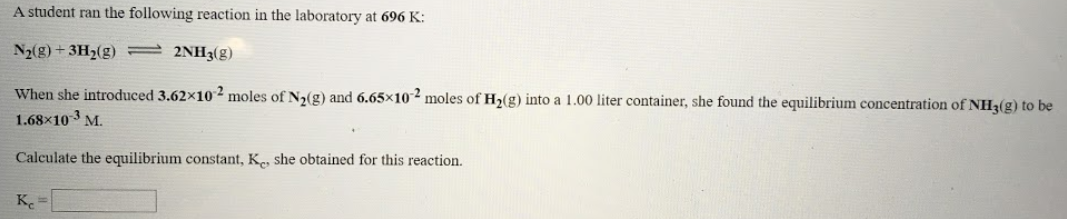# A student ran the following reaction in the laboratory at 696 K: N2(g) + 3H2(g) ⇌ 2NH3(g) When she introduced 3.62X10^-2 moles of N2(g) and 6.65X10^-2 moles of H2(g) into a 1.00 liter container, she found the equilibrium concentration of NH3(g) to be 1.68x10^-3 M. Calculate the equilibrium constant, Kc, she obtained for this reaction.